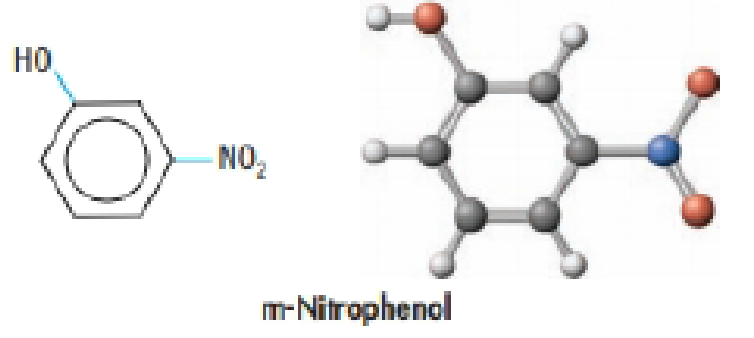Chapter 16, Problem 93GQ

Chapter
Section
Textbook Problem

m-Nitrophenol, a weak acid, can be used as a pH indicator because it is yellow at pH above 8.6 and colorless at pH below 6.8. If the pH of a 0.010M solution of the compound is 3.44, calculates its pKa.Interpretation Introduction

Interpretation:

The pKa value has to be calculated.

Concept introduction:

Equilibrium constants:

The equilibrium constant is used to quantitative measurement of the strength of the acid and bases in the water.

Ka is an acid constant for equilibrium reactions.

HA + H2OH3O++ A-Ka[H3O+][A-][HA]

Kb is a base constant for equilibrium reaction.

BOH + H2OB++ OH-Ka[B+][OH-][BOH]

Explanation

pKa of m-Nitrophenol solution is 4.87.

Let’s calculate the hydronium ion concentration.

pH = -log [H3O+]the given pH = 3.44[H3O+]  = 10-3.44[H3O+]  =  3.6×10-4M

The equilibrium chemical reaction is as follows.

C6H4NO2OH (aq) + H2O(l) C6H4NO2O- (aq) + H3O+(aq)

The equilibrium expression:

Ka[C6H4NO2O-][H3O+][C6H4NO2OH]

Enter the ICE table the concentrations before equilibrium is established, the change that occurs as the reaction proceeds to equilibrium and the concentrations when equilibrium has been achieved.

The given concentration =0.010 M

C6H4NO2OH (aq) + H2O(l) C6H4NO2O- (aq) + H3O+(aq)I         0

Still sussing out bartleby?

Check out a sample textbook solution.

See a sample solution

The Solution to Your Study Problems

Bartleby provides explanations to thousands of textbook problems written by our experts, many with advanced degrees!

Get Started